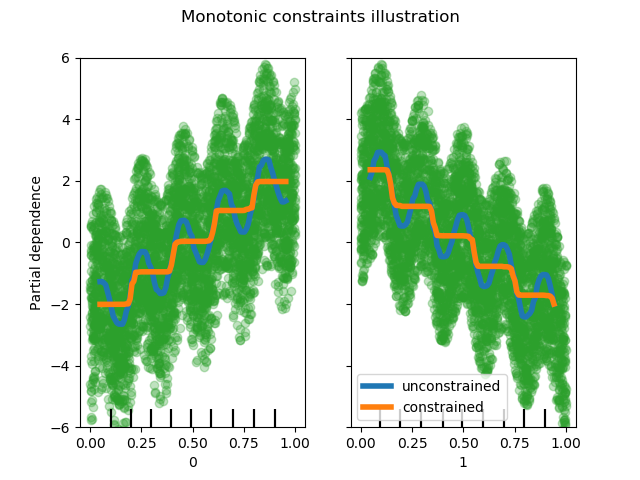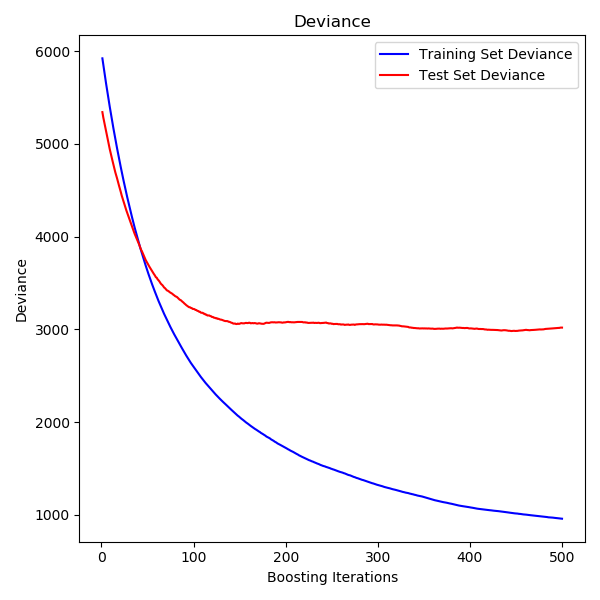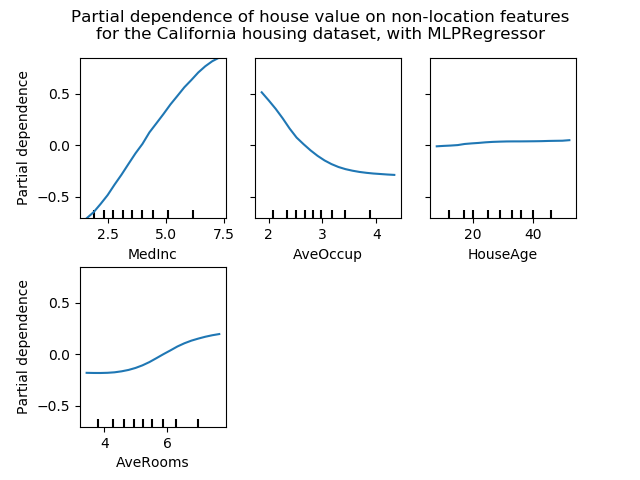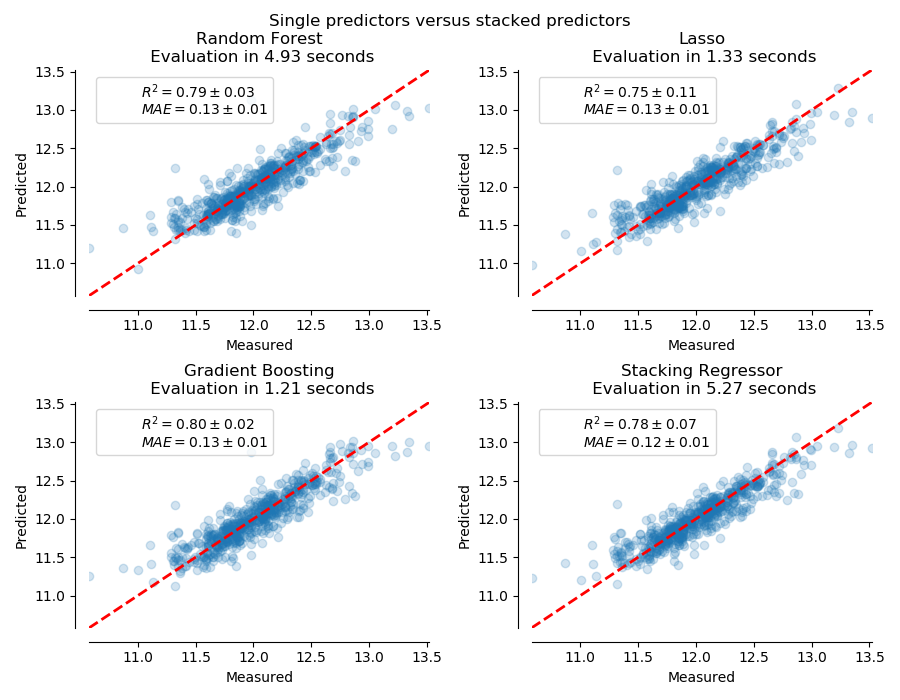class sklearn.ensemble.HistGradientBoostingRegressor(loss='least_squares', *, learning_rate=0.1, max_iter=100, max_leaf_nodes=31, max_depth=None, min_samples_leaf=20, l2_regularization=0.0, max_bins=255, monotonic_cst=None, warm_start=False, early_stopping='auto', scoring='loss', validation_fraction=0.1, n_iter_no_change=10, tol=1e-07, verbose=0, random_state=None)

[源码]

>>> # explicitly require this experimental feature>>> from sklearn.experimental import enable_hist_gradient_boosting  # noqa>>> # now you can import normally from ensemble>>> from sklearn.ensemble import HistGradientBoostingClassifier

0.21版本新功能。

loss {‘least_squares’, ‘least_absolute_deviation’, ‘poisson’}, optional (default=’least_squares’)

learning_rate float, optional (default=0.1)

max_iter int, optional (default=100)

max_leaf_nodes int or None, optional (default=31)

max_depth int or None, optional (default=None)

min_samples_leaf int, optional (default=20)

l2_regularization float, optional (default=0)
L2正则化参数。使用0表示不正则化(默认)。
max_bins int, optional (default=255)

monotonic_cst array-like of int of shape (n_features), default=None

warm_start bool, optional (default=False)

early_stopping ‘auto’ or bool (default=’auto’)

scoring str or callable or None, optional (default=’loss’)

validation_fraction int or float or None, optional (default=0.1)

n_iter_no_change int, optional (default=10)

tol float or None, optional (default=1e-7)

verbose int, optional (default=0)

random_state int, np.random.RandomStateInstance or None, optional (default=None)

n_iter_ int

n_trees_per_iteration_ int

train_score_ ndarray, shape (n_iter_+1,)

validation_score_ ndarray, shape (n_iter_+1,)

>>> # To use this experimental feature, we need to explicitly ask for it:>>> from sklearn.experimental import enable_hist_gradient_boosting  # noqa>>> from sklearn.ensemble import HistGradientBoostingRegressor>>> from sklearn.datasets import load_diabetes>>> X, y = load_diabetes(return_X_y=True)>>> est = HistGradientBoostingRegressor().fit(X, y)>>> est.score(X, y)0.92...

fit(X, y[, sample_weight]) 拟合梯度助推模型。
get_params([deep]) 获取这个估计器的参数。
predict(X) 预测X的值。
score(X, y[, sample_weight]) 返回预测的决定系数R^2。
set_params(**params) 设置这个估计器的参数。
__init__(loss='least_squares', *, learning_rate=0.1, max_iter=100, max_leaf_nodes=31, max_depth=None, min_samples_leaf=20, l2_regularization=0.0, max_bins=255, monotonic_cst=None, warm_start=False, early_stopping='auto', scoring='loss', validation_fraction=0.1, n_iter_no_change=10, tol=1e-07, verbose=0, random_state=None)

[源码]

fit(X, y, sample_weight=None)

[源码]

X array-like of shape (n_samples, n_features)

y array-like of shape (n_samples,)

sample_weight array-like of shape (n_samples,) default=None

self object
get_params(deep=True)

[源码]

deep bool, default=True

params mapping of string to any

predict(X)

[源码]

X array-like, shape (n_samples, n_features)

y ndarray, shape (n_samples,)

score(X, y, sample_weight=None)

X array-like of shape (n_samples, n_features)

y array-like of shape (n_samples,) or (n_samples, n_outputs)
X的值
sample_weight array-like of shape (n_samples,), default=None

score float
R^2 of self.predict(X) wrt. y.

set_params(**params)

[源码]

**params dict

self object